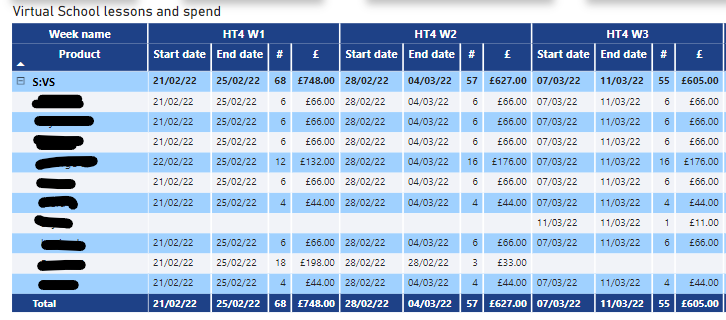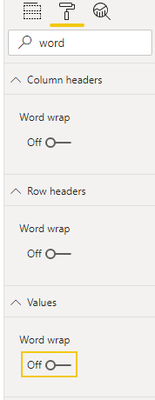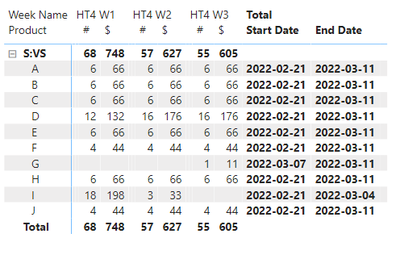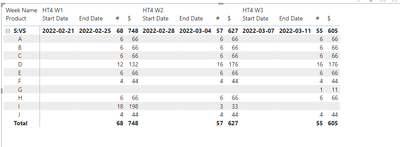cancel
Showing results for
Did you mean:New Member

## Power BI Matrix table - not to subtotal specific data

Hi, firstly apologies for the way this is described, not quite 100% sure how to explain what I require and if its possible but somebody may easily know the answer!

I have a spreadsheet of data that summarises people attending a service and counts their sessions and the cost on a weekly basis. I would like to add two extra columns to this table with the first date and last date of charges. However, when I add this data in (from the same spreadsheet) - it summarises it based on the column headers and repeats the information for every week.

Copy of the matrix table is below before the date field is added.Before date is added

Essentially I want the end and start date to be only added once and not repeated in each week over and over again.After date is added

Hope I've explained this ok and thank you in advance!

1 ACCEPTED SOLUTIONCommunity Support

Hi @phileastman ,

I think you want to show start date and end date only once instead of expanding all week. As far as I know, you add [start date] and [end date] in matrix value field. As I mentioned above, Power BI will expand the column in each column level. So Power BI doesn't support your requirement.

Here I have a workaround that you can try to show min start date and max end date in subtotal. You just need to turn off word wrap in format and then reduce the width  of the column which you don't need to 0.Measure:

``````Start Date =
IF(ISINSCOPE('Table'[Type]), CALCULATE(MIN(DimDate[Start date]),ALLEXCEPT('Table','Table'[Product],'Table'[Type])),BLANK())``````
``````End Date =
IF(ISINSCOPE('Table'[Type]), CALCULATE(MAX(DimDate[End date]),ALLEXCEPT('Table','Table'[Product],'Table'[Type])),BLANK())``````

Result is as below.Best Regards,
Rico Zhou

If this post helps, then please consider Accept it as the solution to help the other members find it more quickly.

3 REPLIES 3Community Support

Hi @phileastman ,

Do you want to show start date and end date value only once instead of repeating in all Products each week? As far as I know, you add [start date] and [end date] in matrix value field, so it will repeat in all rows each week.

You can try to create measures by IF and ISINSCOPE function.

``````Start Date =
IF(ISINSCOPE('Table'[Type]),BLANK(),IF(ISINSCOPE('Table'[Product]),CALCULATE(MAX(DimDate[Start date]))))``````
``````End Date =
IF(ISINSCOPE('Table'[Type]),BLANK(),IF(ISINSCOPE('Table'[Product]),CALCULATE(MAX(DimDate[End date]))))``````

Result is as below.Best Regards,
Rico Zhou

If this post helps, then please consider Accept it as the solution to help the other members find it more quickly.New Member

Hi Rico

Thank you for the reply, it's greatly appreciated. If possible I would like the start and end date to be for each individual e.g. A, B, C, D but only shown once for the earliest and latest dates rather than each week that it shows by default:Community Support

Hi @phileastman ,

I think you want to show start date and end date only once instead of expanding all week. As far as I know, you add [start date] and [end date] in matrix value field. As I mentioned above, Power BI will expand the column in each column level. So Power BI doesn't support your requirement.

Here I have a workaround that you can try to show min start date and max end date in subtotal. You just need to turn off word wrap in format and then reduce the width  of the column which you don't need to 0.Measure:

``````Start Date =
IF(ISINSCOPE('Table'[Type]), CALCULATE(MIN(DimDate[Start date]),ALLEXCEPT('Table','Table'[Product],'Table'[Type])),BLANK())``````
``````End Date =
IF(ISINSCOPE('Table'[Type]), CALCULATE(MAX(DimDate[End date]),ALLEXCEPT('Table','Table'[Product],'Table'[Type])),BLANK())``````

Result is as below.Best Regards,
Rico Zhou

If this post helps, then please consider Accept it as the solution to help the other members find it more quickly.